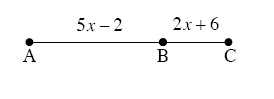### Home > GC > Chapter Ch1 > Lesson 1.2.1 > Problem1-56

1-56.

The distance along a straight road is measured as shown in the diagram below. If the distance between towns $A$ and $C$ is $67$ miles, find the distance between towns $A$ and $B$. Homework Help ✎$5x − 2 + 2x + 6 = 67$